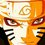# Olympiad Proof Problem - Day 3

This is the third proof problem of the series "Olympiad Proof Problems". Try this problem and post your working.

Find the maximum value of the expression $ab + bc + cd + da$, subject to the conditions $abcd = 4$ and $a^2 + b^2 + c^2 + d^2 =10$.

Details and Assumptions :

• $a$, $b$, $c$ and $d$ are positive real numbers

Try more proof problems at Olympiad Proof Problems.

EDIT: When dealing with inequalities, find out the condition of equality and check whether the equality condition satisfies the given constraints.Note by Surya Prakash
5 years, 8 months ago

This discussion board is a place to discuss our Daily Challenges and the math and science related to those challenges. Explanations are more than just a solution — they should explain the steps and thinking strategies that you used to obtain the solution. Comments should further the discussion of math and science.

When posting on Brilliant:

• Use the emojis to react to an explanation, whether you're congratulating a job well done , or just really confused .
• Ask specific questions about the challenge or the steps in somebody's explanation. Well-posed questions can add a lot to the discussion, but posting "I don't understand!" doesn't help anyone.
• Try to contribute something new to the discussion, whether it is an extension, generalization or other idea related to the challenge.

MarkdownAppears as
*italics* or _italics_ italics
**bold** or __bold__ bold
- bulleted- list
• bulleted
• list
1. numbered2. list
1. numbered
2. list
Note: you must add a full line of space before and after lists for them to show up correctly
paragraph 1paragraph 2

paragraph 1

paragraph 2

[example link](https://brilliant.org)example link
> This is a quote
This is a quote
    # I indented these lines
# 4 spaces, and now they show
# up as a code block.

print "hello world"
# I indented these lines
# 4 spaces, and now they show
# up as a code block.

print "hello world"
MathAppears as
Remember to wrap math in $$ ... $$ or $ ... $ to ensure proper formatting.
2 \times 3 $2 \times 3$
2^{34} $2^{34}$
a_{i-1} $a_{i-1}$
\frac{2}{3} $\frac{2}{3}$
\sqrt{2} $\sqrt{2}$
\sum_{i=1}^3 $\sum_{i=1}^3$
\sin \theta $\sin \theta$
\boxed{123} $\boxed{123}$

Sort by:

I have a fairly long proof for this but hopefully, it is correct. Note that $ab+bc+cd+da=(a+c)(b+d)$.

WLOG, we may assume that $ac \geq bd$. From this, we have $a^2c^2 \geq abcd=4$ and hence $ac \geq 2$.

Now, using the AM-GM inequality, we have

$10=(a^2+c^2)+(b^2+d^2) \geq 2ac+2bd=2ac+\frac{8}{ac}.$

Solving the inequality $2ac+\frac{8}{ac} \leq 10$, we get $1 \geq ac \leq 4.$ Combining with the result $ac \geq 2$ obtained above, we get $2 \leq ac \leq 4$.

Setting $m=a^2+c^2$ and $n=ac$ ($m \geq 2n,\, 2 \leq n \leq 4$). Under this substitution, we have

$(a+c)^2=m+2n,\quad (b+d)^2=b^2+d^2+2bd=10-m+\frac{8}{n}.$

Therefore,

$(a+c)^2(b+d)^2=(m+2n)\left( 10-m+\frac{8}{n}\right) =\left(n+5+\frac{4}{n}\right)^2 -\left(m+n-5-\frac{4}{n}\right)^2.$

Let's consider two cases:

Case 1: $3n^2-5n+4 \geq 0$

In this case, we have

$m+n-5-\frac{4}{n} \geq 3n-5-\frac{4}{n}=\frac{3n^2-5n-4}{n} \geq 0.$

Therefore,

\begin{aligned} (a+c)^2(b+d)^2 & \leq \left(n+5+\frac{4}{n}\right)^2 -\left(3n-5-\frac{4}{n}\right)^2=8\left[n(5-n)+4\right] \\ &\leq 8 \left\{ \left[ \frac{n+(5-n)}{2}\right]^2+4\right\}=82.\end{aligned}

Equality holds when $m=2n,\, n=\frac{5}{2}, a^2+b^2+c^2+d^2=10$ and $abcd=4$. This can be attained for example when $a=c=\sqrt{\frac{5}{2}},\quad b=\frac{\sqrt{205}+3\sqrt{5}}{10}, \quad d=\frac{\sqrt{205}-3\sqrt{5}}{10}$.

Case 2: $3n^2 - 5n + 4 < 0$

In this case, we have $2 \leq n < \frac{5+\sqrt{73}}{6}$. Since the function $f(n)=n+\frac{4}{n}+5$ is increasing for $n \ge 2$, we get

$f(n)

And thus, it follows that

$(a+c)^2(b+d)^2 \leq \left(n+5+\frac{4}{n}\right)^2 <\left(\frac{10+2\sqrt{73}}{3}\right)^2<82.$

From this, it follows that the maximum value for $(a+c)(b+d)=\sqrt{82}.$

Proof No.2 (Way shorter)

Once again, $ab+bc+cd+da=(a+c)(b+d)$. We have

$(a+c)(b+d)=\sqrt{(a^2+c^2+2ac)(b^2+d^2+2bd)}$

$= \sqrt{(a^2+c^2)(b^2+d^2)+2ac(b^2+d^2)+2bd(a^2+c^2)+4abcd}$

$\leq \sqrt{\left(\frac{a^2+b^2+c^2+d^2}{2}\right)^2 +2(ab+cd)(ad+bc)+16}$

$\leq \sqrt{25+2\left(\frac{ab+bc+cd+da}{2}\right)^2+16}$

$=\sqrt{41+\frac{1}{2} (a+c)^2 (b+d)^2}$

Solving, we have

$(a+c)(b+d) \leq \sqrt{41+\frac{1}{2} (a+c)^2 (b+d)^2}$

$(a+c)^2 (b+d)^2 \leq 41+\frac{1}{2} (a+c)^2 (b+d)^2$

$\frac{1}{2} (a+c)^2 (b+d)^2 \leq 41$

$(a+c)(b+d) \leq \sqrt{82}$

- 5 years, 8 months ago

@Surya Prakash , the difficulty spiked dramatically here. Perhaps a bit too much. But please, continue posting olympiad questions like this.

- 5 years, 8 months ago

You made it too much lengthy.

- 5 years, 8 months ago

I do believe I have another proof which is much shorter. I can't believe I didn't think about this.

Once again, $ab+bc+cd+da=(a+c)(b+d)$. We have

$(a+c)(b+d)=\sqrt{(a^2+c^2+2ac)(b^2+d^2+2bd)}$

$= \sqrt{(a^2+c^2)(b^2+d^2)+2ac(b^2+d^2)+2bd(a^2+c^2)+4abcd}$

$\leq \sqrt{\left(\frac{a^2+b^2+c^2+d^2}{2}\right)^2 +2(ab+cd)(ad+bc)+16}$

$\leq \sqrt{25+2\left(\frac{ab+bc+cd+da}{2}\right)^2+16}$

$=\sqrt{41+\frac{1}{2} (a+c)^2 (b+d)^2}$

Solving, we have

$(a+c)(b+d) \leq \sqrt{41+\frac{1}{2} (a+c)^2 (b+d)^2}$

$(a+c)^2 (b+d)^2 \leq 41+\frac{1}{2} (a+c)^2 (b+d)^2$

$\frac{1}{2} (a+c)^2 (b+d)^2 \leq 41$

$(a+c)(b+d) \leq \sqrt{82}$

- 5 years, 8 months ago

@Surya Prakash , Is this a better proof?

- 5 years, 8 months ago

Awesome way

- 5 years, 8 months ago

Yes this was exactly the way I solved it.

- 5 years, 8 months ago

So you have a shorter solution? Can you please share on Slack if you don't want to put it here?

- 5 years, 8 months ago

Yes I also got √82 and it's correct.

- 5 years, 8 months ago

Using Cauchy Scharw Inequality,

$(a^2 +b^2 + c^2 + d^2)(b^2 + c^2 + d^2 + a^2) \geq (ab+bc+cd+da)^2$

So $10\geq (ab+bc+cd+da)$.

- 5 years, 8 months ago

Using this we get that equality holds when $\dfrac{a}{b} = \dfrac{b}{c} = \dfrac{c}{d} = \dfrac{d}{a}$. Which gives us that$a=b=c=d$. So, from the equation $a^2 + b^2 + c^2 + d^2 =10$, we get that $a=b=c=d=\sqrt{\dfrac{5}{2}}$. This gives us that $abcd=\dfrac{25}{4}$. But $abcd=4$. So your solution is wrong.

- 5 years, 8 months ago

OH! didnt notice that

- 5 years, 8 months ago

You only used the first condition that a^2+b^2+c^2+d^3=10. What about abcd=4?

- 5 years, 8 months ago